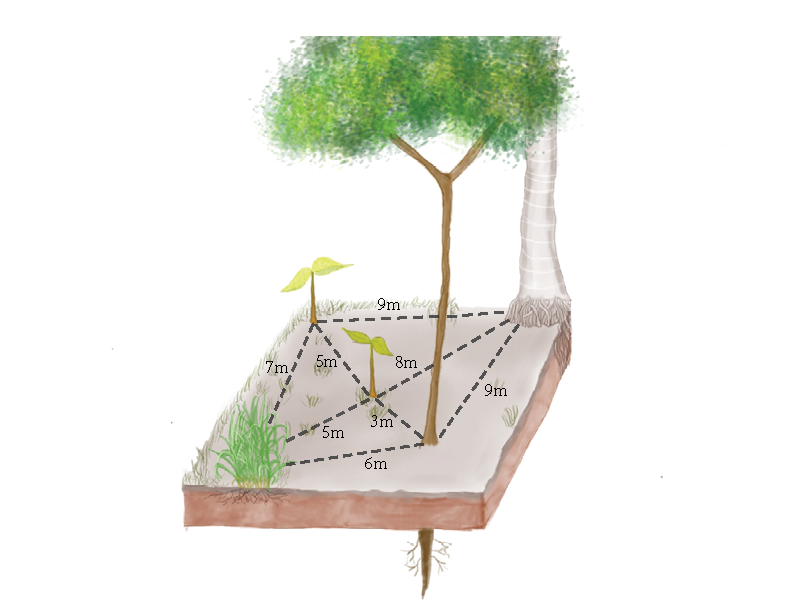# 1. Introduction

Interactions between neighboring plants impact how plants grow, survive, and reproduce. Although interactions occur at the scale of individual plants, the consequences of plant-plant interactions shape population and community structure across terrestrial ecosystems. Plants tend to do worse in single-species neighborhoods than in many-species neighborhoods (Sortibrán et al. 2014), a plant level dynamic that helps explain how plant biodiversity is maintained across ecosystems, from montane deserts (Adler et al. 2010) to tropical rainforests (Comita et al. 2010). Managing plant neighborhoods, from thinning dense stands of trees (Cescatti and Piutti 1998, Lechuga et al. 2017) to planting species that will facilitate their neighbors (Gómez-Aparicio 2009), is a cornerstone of forestry, restoration, and agriculture. The importance of plant-plant interactions across basic and applied ecology points to the need for analyses to quantify how neighborhoods impact plant demography. Such analyses must account for space, as plants interact more with closer neighbors than with neighbors further away (Fig.1).Figure 1: Spatial structure of a plant neighborhood. The seedling in the center of the plot experiences a range of plant-plant interactions, depending on the neighbor’s species identity, plant size, and physical distance.

The objective of this case study is to illustrate how to fit spatially-explicit models for plant-plant interactions in Stan. Using multiple examples of plant demographic rates, including growth, survival, and recruitment, we address the following objectives:

1. Develop models that account for non-linear relationships between interactions strength and pairwise distances between neighboring plants:

Explicitly estimating distance-decay parameters in neighborhood models enables greater flexibility and opportunities for novel inference. We use simulated data to demonstrate Stan’s ability to fit non-linear models for plant-plant interactions.

2. Efficient strategies for modeling pairwise interactions between many plants:

Spatially-explicit models require accounting for pairwise interactions between neighbors, leading to large matrices. Every individual element added to a pairwise distance matrix increases matrix size following a series of square numbers. However, not all plants represented in the pairwise matrix interact, so this kind of data accumulates many zeroes, which creates a sparse matrix. We will demonstrate how to efficiently handle sparse matrices in plant neighborhood models, using Stan’s segment function to eliminate zero-valued elements from computation.

3. For hierarchical neighborhood models, we explore the costs and benefits of the non-centered parameterization for model convergence:

Hierarchical structures, including random effects that represent spatial and temporal covariance, are common in ecological data. We show how plant-plant interaction models can incorporate random effects and then explore the consequences of centered and non-centered parameterization (Betancourt and Girolami 2013) for model-fitting.

# 2. Environment

This case study uses Stan version 2.19.1 and the following R packages.

  library(rstan)
library(ggplot2)
library(bayesplot)
library(rethinking)
library(gridExtra)
library(kableExtra)

# 3. Simulation: Effect of neighbor plants on growth

We start by simulating a spatially explicit data set of plant growth and then fitting a neighborhood model. We model the growth of stationary plants as a function of their intrinsic growth (growth in isolation) and their local neighborhood characteristics (i.e., neighbor size and proximity; Eq. 3).

$\begin{split} growth\sim normal(\mu,\sigma ^2)\\ \ \mu_j = \alpha + \beta \textrm{s}_0 + a_3 \sum\limits_{j=1}^n \frac {\textrm{size}_{j}^{a_1}} {\textrm{distance}_{j}^2 a_2} \quad Eq. 3 \end{split}$

Where $$\alpha$$ is the intercept, $$\beta$$ is the growth rate of plants depending on their initial size, $$\mathit{\textrm{s}_0}$$ is the initial size of the target plant. Size, and distance are the matrices containing the size and distance of neighbors, and $$\mathit{a_1}$$, $$\mathit{a_2}$$ and $$\mathit{a_3}$$ are parameters describing the relationship of growth with the neighbors’s size and distance.

## 3.1 Data simulation

The generative model includes two predictor variables (Fig.2); the size of plants at time t and t+1, and a spatial component; the x and y coordinates for each individual:

N=500
size_t0=rnorm(N,mean=10, sd=1)
size_t1=rnorm(N, mean=35,sd=1)

x=runif(N,min=0,max=14)
y=runif(N,min=0,max=14)
distmat=as.matrix(dist(cbind(x,y),method="euclidean"))
sizemat=matrix(rep(size_t0,500),nrow=500,ncol=500,byrow=T)